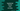# How to iterate over an array in TypeScript## Introduction :

Iterating over an array is one of the most commonly faced problems in any programming language. In typescript, we have multiple ways to iterate an array. Using loops and using its inbuilt method forEach, we can iterate through the array elements. In this tutorial, I will show you different ways to do it with examples.

## Using a for loop :

This is the most straightforward approach. The length property of an array variable is its length and the index of the first item is 0, second item is 1, etc. i.e. using a for loop, we can iterate from 0 to length - 1 as the current index and access each element for that specific index.

``````let arr = [1, 2, 3, 4, 5];

for (var i = 0; i < arr.length; i++) {
console.log(arr[i]);
}``````

It will print the below output :

``````1;
2;
3;
4;
5;``````

## Using for..in loop :

We can also iterate through the array elements using a for..in loop. It returns the index on each iteration. For example :

``````for (var i in arr) {
console.log(arr[i]);
}``````

It will print the same output.

## Using for..of loop :

As explained in the above example, for..in loop iterates through the array items and returns the index for each element. Instead, we can use for..of loop that iterates through the items and returns the values.

``````for (var item of arr) {
console.log(item);
}``````

We can access the items directly without the index. This one is better than the for..in loop if you need only the values.

## Using forEach :

forEach is an inbuilt method. It is short, and we can access each value or both index and value of an array.

Example to get only values :

``````let arr = [11, 12, 13, 14, 15];

arr.forEach((e) => {
console.log(e);
});``````

Output :

``````11;
12;
13;
14;
15;``````

Example to get both index and value :

``````let arr = [11, 12, 13, 14, 15];

arr.forEach((e, i) => {
console.log(`arr[\${i}] : \${e}`);
});``````

Output :

``````arr : 11
arr : 12
arr : 13
arr : 14
arr : 15
``````